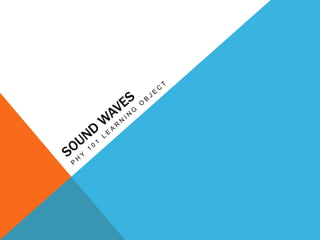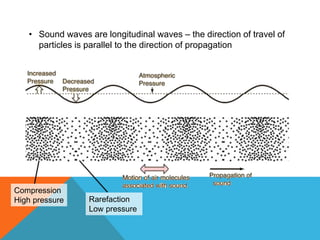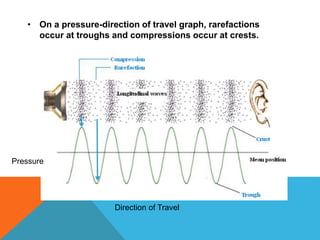Successfully reported this slideshow.

# LO4 - Sound Waves×

# LO4 - Sound Waves

This powerpoint presentation looks at some properties of sound waves and explains the relationship between displacement and pressure of sound waves with a practice problem at the end.

This powerpoint presentation looks at some properties of sound waves and explains the relationship between displacement and pressure of sound waves with a practice problem at the end.

### LO4 - Sound Waves

1. 1. • Sound waves are longitudinal waves – the direction of travel of particles is parallel to the direction of propagation Compression High pressure Rarefaction Low pressure
2. 2. • On a pressure-direction of travel graph, rarefactions occur at troughs and compressions occur at crests. Pressure Direction of Travel
3. 3. SO WHAT IS THE RELATIONSHIP BETWEEN DISPLACEMENT AND PRESSURE??
4. 4. • Consider a sound wave of single frequency • The displacement and pressure graphs are shown on the right
5. 5. A FEW OBSERVATIONS • When the displacement is at the equilibrium position (y = 0), the pressure is at its highest • When the displacement is the greatest (y = 10), the pressure is 0
6. 6. WHY??? • When the pressure is high (at compressions), particles are pushed into the area from both left and right • Displacement is positive on the right and negative on the left • This occurs when the particle passes through zero, where the pressure is maximized
7. 7. • When the pressure is low (at rarefactions), particles moves away on both left and right • Particle in the area is stationary and can move left or right • This happens when the displacement is at its crest or trough
8. 8. RECAP… • When the displacement is at its greatest, pressure is zero • When the displacement is zero, pressure is at its greatest. • If the displacement follows a sine function, the pressure changes like a cosine function • i.e. displacement and pressure are π/2 radians out of phase Displacement Pressure Π/2 rad
9. 9. FOOD FOR THOUGHT Question: Imagine an alien from a planet called Pressurnet came to visit Earth. You wanted to approach the alien but he is sensitive to pressure changes. He is standing 10.378 meters away from you when you decided to call out to him. The displacement amplitude of the your sound (as it reached the alien) is 0.3405 μm and the frequency is 1000 Hz. [assume the displacement follows a sine function] a. What is the pressure of your voice as it reaches the alien? b. If the alien runs away when it is subjected to pressure higher than 0.5 mPa, will he run away when you call out to him?
10. 10. Using equation 15-11 on page 427 of the textbook, • Δpm = Bksm • B = 1.01 x 105 Pa • k = 2*π/λ =2*π*f/v (since v = f*λ, i.e. λ = v/f) • Therefore, • Δpm = (B*2*π*f*sm)/v • = (1.01 x 105 Pa * 2 * π *1000 * 0.3405 μm)/(343 m/s) • = 0.00063 Pa = 0.63 mPa
11. 11. WRAPPING UP… a. The pressure of the sound as it reaches the alien is approximately 0.63 mPa. a. Since 0.63 mPa > 0.5 mPa, the alien will run away when you call out to him.
12. 12. THANKS FOR WATCHING :]
13. 13. REFERENCES Hawks, R., Iqbal, J., Mansour, F., Milner-Bolotin, M., & Williams P. 2014. Physics for Scientists and Engineers: An Interactive Approach (1st ed.) Nelson. Nave, R. Sound. HyperPhysics. Retrieved from http://hyperphysics.phy- astr.gsu.edu/hbase/sound/tralon.html [accessed 20 Feb 2015] Sound Reflection. 2014. TutorVista. Retrieved from http://physics.tutorvista.com/waves/sound-reflection.html [accessed 20 Feb 2015]